# Thursday  February 9

•  Now... what about finding all the reflective and rotational symmetries of a single figure?

• Symmetries of playing card.... classify the cards by having same symmetries.

Notice symmetry of clubs, diamonds, hearts, spades

Symmetries of an equilateral triangle:

•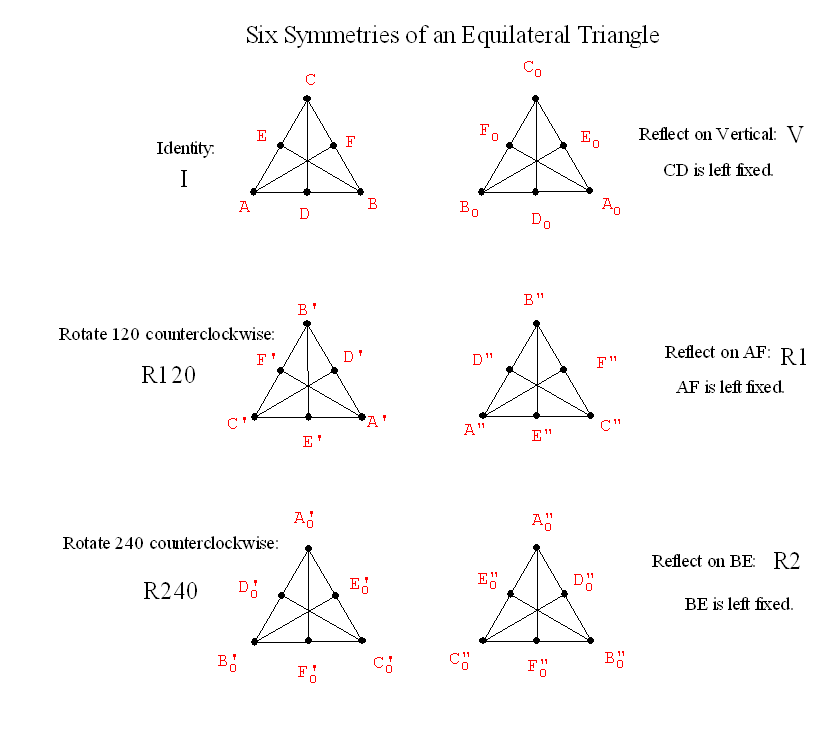• Combining transformations to give new symmetries:
Example: Let V denote reflection across the line that is the vertical altitude of the equilateral triangle.

And consider a second symmetry, called R=R120, which will rotate the equilateral triangle counterclockwise about its center O by 120 degrees.

We first perform V to the figure and then perform R to the figure.
We will denote this R*V
... meaning V followed by R.

The resulting transformation also leaves the equilateral  covering the same position in which it started, and....

R*V  =   R2.

What about other products?  This gives a  "product" for symmetries.
If S and R are any symmetries of a figure then S*R is also a symmetry of the figure.

A "multiplication" table for Symmetries: check these!
 * Id R120 R240 V G=R1 H=R2 Id I R120 R240 R2 R240 I R1 V R1 R2 I R120 G=R1 I H=R2 I
R240*V = R1  why?
This "multiplicative" structure  is called the Group of symmetries of the equilateral triangle.

Given any figure we can talk about the group of its symmetries.
Does a figure always have at least one symmetry? .....

Yes... The Identity symmetry.
Such a symmetry is called the trival symmetry.

So we can compare objects for symmetries....
how many?
does the multiplication table for the symmetries look the same in some sense?

What about the symmetries of a Frieze Pattern on a Strip....
Translations and  Reflections

...|p|q|p|q|p|q|p|q|p|q|p|...

This pattern has vertical axes for reflective symmetries between each p and q... and a translation symmetry taking each letter to the next letter of the same type... and also twice as far, and three times as far , and more....

The same is true for the following pattern:

...|d|b|d|b|d|b|d|b|d|b|d|...

Notice the difference -180 degree Rotations and Translations are symmetries for the next pattern.
...|p|p|p|p|p|...
...|d|d|d|d|d|...

The following pattern also has glide reflection symmetry, for example taking p to b and q to d, etc.
It also has a 180 degree rotational symmetry with center midway between the vertical reflection axes and the letter p and d or q and b.

...|p|q|p|q|p|q|p|q|p|q|p|...
...|d|b|d|b|d|b|d|b|d|b|d|...

What are the possible symmetries of a Frieze Pattern on a Strip....

Show video on symmetries from FAPP.

Do activity on recognizing symmetries in frieze and planar patterns.

Based on the group of symmetries for these patterns,
there are seven possible distinct types of frieze or
Border Patterns: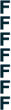translation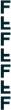horizontal reflection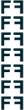vertical reflection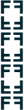reflection + reflection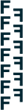glide reflection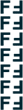rotation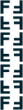reflection + glide reflection

What about the symmetries of a tiling? There are 17 distinct symmetry groups for tiling the plane. They can be described by the following diagrams indicating the symmetries of the figures  as below: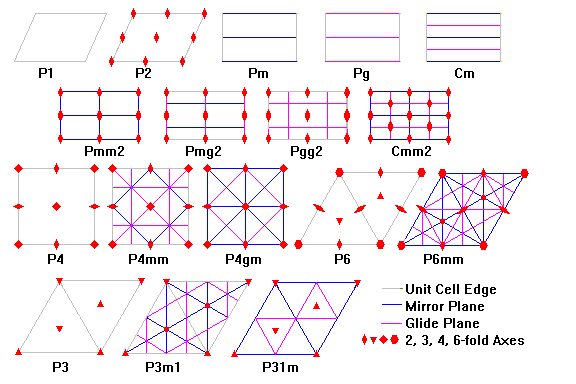or with figures as illustrated by the following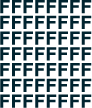translations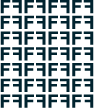reflections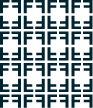reflections + reflections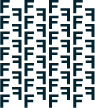glide reflections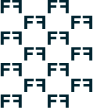reflections + glide reflections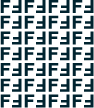rotations (2)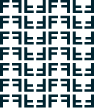reflections + rotations (2)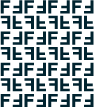rotations (2) + glide reflections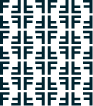rotations (2) + reflections + reflections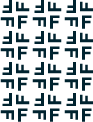rotations (4)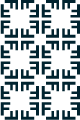reflections + rotations (4)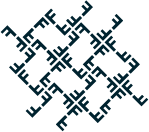rotations (4) + reflections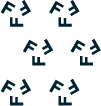rotations (3)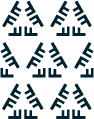reflections + rotations (3)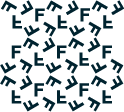rotations (3) + reflectionsrotations (6)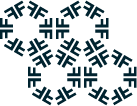reflections + rotations (6)